Thinking

# Should the Pythagorean theorem be renamed the Thalean theorem?

Thales may have known the famous theorem perhaps as much as half a century before Pythagoras.

Key Takeaways
• The Greeks believed that geometry and philosophy were inextricably linked.
• The mathematician Thales is credited with several geometrical propositions.
• It is plausible that his investigations into triangles led him to a visual proof of the Pythagorean theorem decades before Pythagoras. Pythagoras or his followers might have proved the theorem subsequently.

Among my colleagues working in ancient science, mathematics, and philosophy, the conventional view is that Pythagoras (c. 570-495 BCE) has been discredited as being the discoverer of the famous theorem bearing his name because the reports connecting him with it are judged too late to be secure testimony. My argument here is that the theorem might very well have been “visualized” by Thales (c. 625-545 BCE) and hence grasped already by the middle of the 6th century, c. 550 BCE, pushing back the Greek discovery by as much as half a century or more.

## Proof: the cosmos is constructed of right triangles

To get the background for this story, I direct the readers to my earlier essays in which I tried to show that Plato’s Timaeus (mid-4th century BCE) memorializes what I call the “lost narrative” — the connection of philosophy and geometry in Thales and Pythagoras. Plato’s Timaeus promulgates the view that the right triangle is the fundamental geometrical figure. The logic is as follows:

All objects have surfaces, and every surface can be dissected into triangles (Figure 1). Inside every triangle are two right triangles (Figure 2).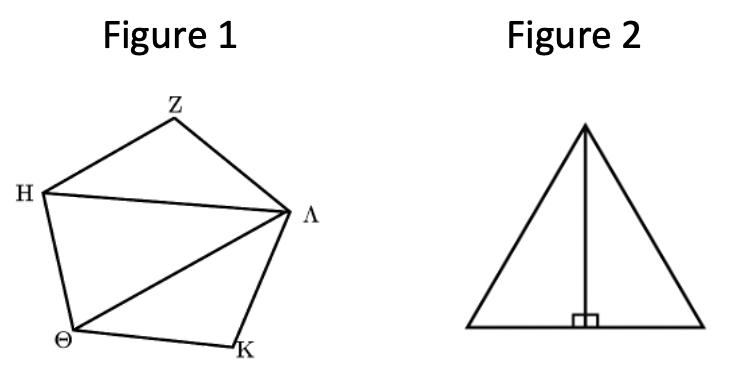If we continue to divide from the right angle, we can create two similar right triangles, isosceles and scalene (Figure 3).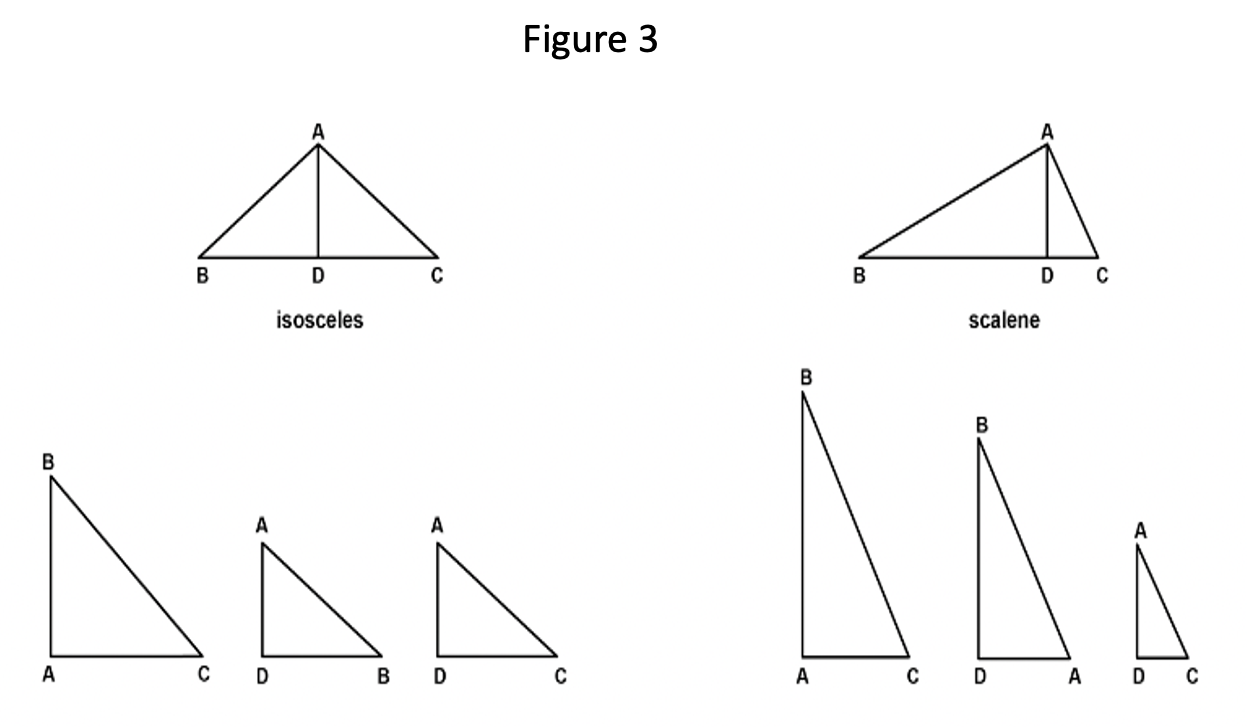This division into smaller and smaller triangles can continue forever (Figure 4). Therefore, the whole cosmos is built out of right triangles!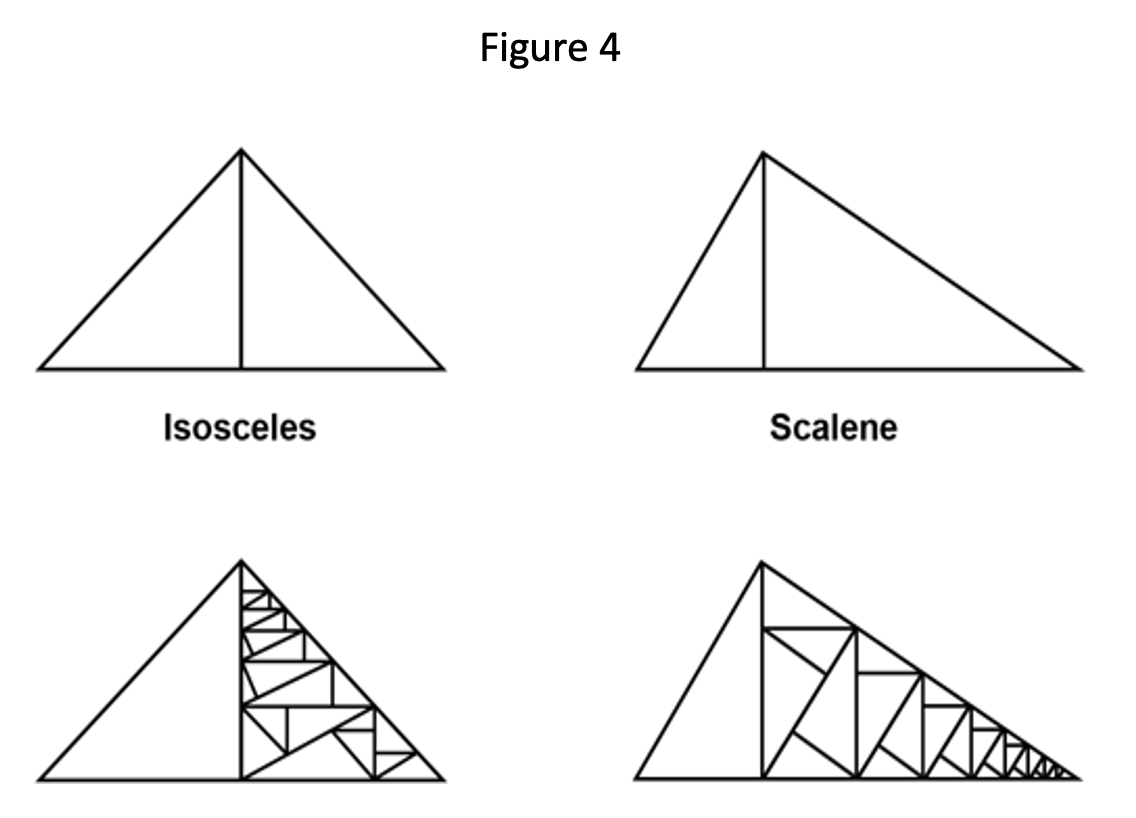Nobody can make such a grand claim without a proof, or line of reasoning, to show that the right triangle is the fundamental geometrical figure. It seems to me — and this is entirely overlooked in the secondary literature — that the proof was the Pythagorean theorem.

But let me emphasize a point that is rarely raised on this Greek problem: which proof? Since we know that there are more than 350 proofs of the Pythagorean theorem, which one was used? Does one of those proofs show that the right triangle is the fundamental geometrical figure of all cosmic appearances?

Yes, it seems to me that the lines of one of two proofs preserved by Euclid VI.31 — the proof by similar right triangles, the so-called enlargement of the Pythagorean theorem — follows just this line of reasoning. Could Thales have visualized the hypotenuse theorem along these lines and Pythagoras (or his followers, the Pythagoreans) proved it later? Perhaps the scholarly consensus has it wrong? Pythagoras may well have proved the theorem as it was already visualized by his older contemporary, Thales.

## Thales’ vision

If Thales visualized it, how exactly?

Among the geometrical propositions credited by name to Thales is the isosceles triangle proposition: if a triangle has two sides of equal length, the angles opposite those sides must be equal. This proposition was pivotal in another geometrical discovery attributed to Thales that every triangle inscribed in a circle on its diameter must be right-angled. Let’s take another look at this diagram.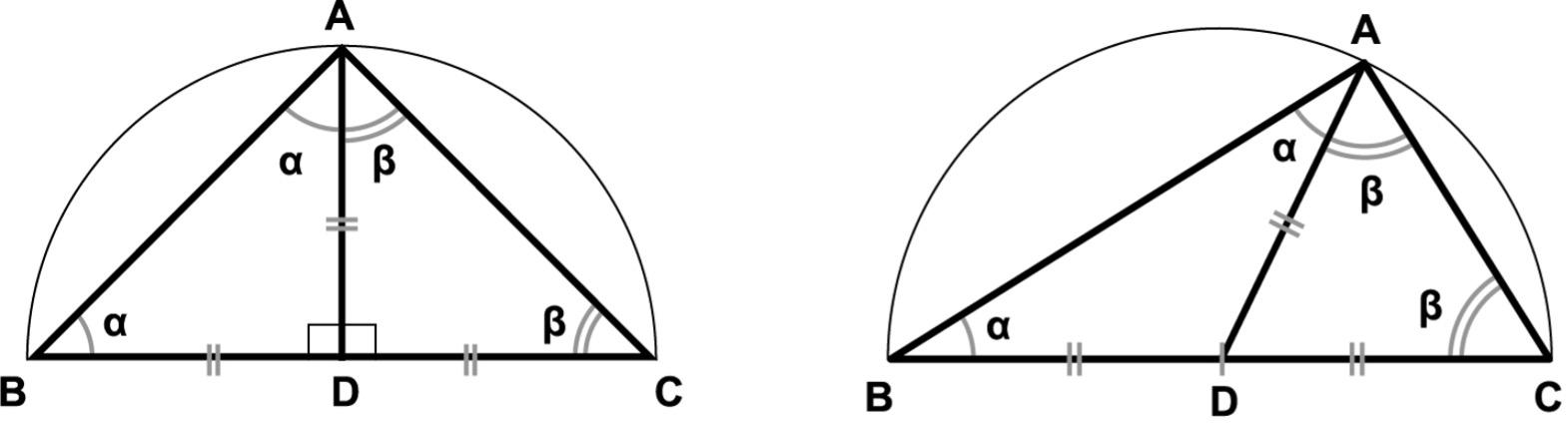As shown above, the isosceles right triangle is on the left, and the scalene right triangle is on the right. When Thales realizes this, he has a way to make countless right triangles for further investigation. He knows the angles in every triangle sum to two right angles (that is, 180°). In the diagram on the left, since BD and AD are both radii of the circle ABC, they must be equal in length, and so angles α and α must be equal. The angle ADB is right, so each angle α must equal half of a right angle. One can see immediately the argument is the same, ceteris paribus, for β, and so β equals half of a right angle. Hence, α + β also equals one right angle, and every triangle inscribed in a circle on its diameter must be right.

Now, had Thales followed this line of thought, he can see inside every right triangle, as they collapse (or expand) by the perpendicular AD from the right angle A to the hypotenuse BC, they do so in a pattern: the square on the perpendicular AD (that is, the square bounded by AD and DC, which are of equal length) is equal in area to the rectangle made by the two parts of BC into which the perpendicular divides the hypotenuse. (Imagine that the second rectangle — in this case, also a square — has length BD and width DC after this latter line segment is “folded” downward.)To see that pattern is to discover the “mean proportional” or “continuous proportion” (BD:AD :: AD:DC).

This is immediately obvious in the case of the isosceles right triangle (shown on the left). Since BD, AD, and DC are all radii of the circle, they must be equal in length, and so the square on AD/DC is equal to the square made by the two parts in which the hypotenuse is divided, BD/DC. For the scalene right triangle, the areal equivalences — that is, the square on AD/DC equals the rectangle on BD/DC (after DC is “folded” downward making the width of the rectangle) — would have to be confirmed empirically, with a compass and ruler.

Now, had Thales noticed this pattern of continuous proportions by which the right triangles collapse (or expand), he might have looked more closely yet and wondered if there were other “mean proportionals” to be discovered. Had he done so, he was in a position to observe that there were indeed two more.

In the scalene right triangle shown below (on the right), the whole hypotenuse (BC) of the largest triangle ABC is to its shortest side (AC) as the hypotenuse (AC) of the smallest triangle ADC is to its shortest side (DC). In other words, BC:AC :: AC:DC.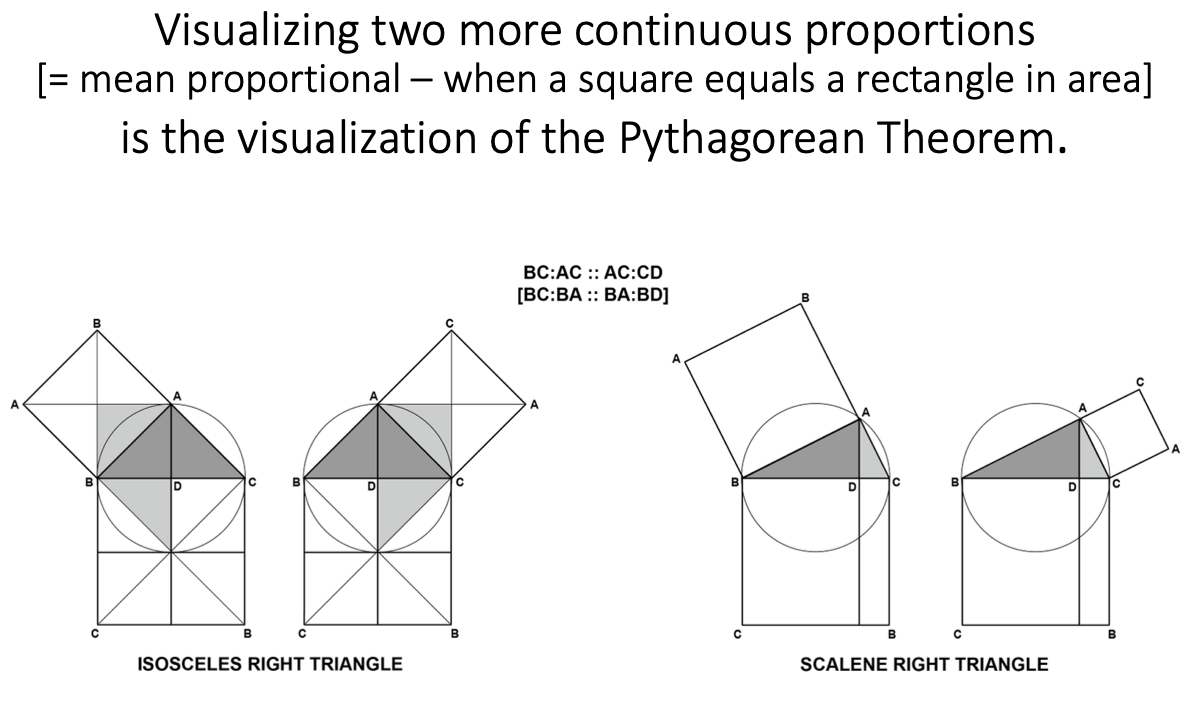In the case of the isosceles right triangle (shown above on the left), the perpendicular divides triangle ABC into two equal smaller triangles, but the continuous proportion still appears: BC:AC :: AC:DC. Geometrically, this means that the square on AC is equal to the figure made by the two parts into which the hypotenuse is divided, which is a rectangle.

Symmetrically, then, on the other side, BC:AB :: AB:BD, hence the square on AB is equal to the rectangle made by the two parts into which the hypotenuse is divided. One can see this areal equivalence immediately in the case of the isosceles right triangle; the scalene right triangle must be measured empirically to confirm.

## The case for Thales’ discovery of the hypotenuse theorem

The visualization of two “mean proportionals” or “continuous proportions” is the visualization of one proof of the Pythagorean theorem. Had Thales followed this line of reasoning, he would have visualized the hypotenuse theorem before the time of Pythagoras and the Pythagoreans. And he would have done so as an unanticipated consequence of searching for and identifying the fundamental geometrical figure — the right triangle — and then looking inside to see what more he might discover.

According to Aristotle, Thales and the earliest philosophers posited a basic underlying nature out of which all things appeared; Thales called it water. Because this underlying unity never perishes, all appearances are only alterations or modifications of water. How does this happen? Might Thales’ explorations in geometry have been to discover the underlying structure of water, and he concluded it was the right triangle? If so, now we can see from Plato’s Timaeus, looking back a century and a half, how the project began of building the cosmos out of right triangles.

Related

Up Next